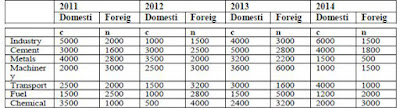### Data Interpretation Quiz

Direction(01-05): Study the given table carefully to answer the following questions:

Following table shows the investment (In Rs. Crore) in various sectors in different years1). What is the difference between the total domestic investment and the total foreign investment in the year 2011?
a) Rs.6400 Crore
b) Rs.6200 Crore
c) Rs.6600 Crore
d) Rs.7000 Crore
e) Rs.7100 Crore

2). What is the ratio of the total investment in Metals to that in Machinery?
a) 135 : 302
b) 24 : 49
c) 2 : 4
d) 197 : 226
e) 123 : 233

3). What is the average domestic investment in the year 2014? (You are not expected to calculate the exact value?
a) Rs.2814.28 Crore
b) Rs.2519.75 Crore
c) Rs.2234.82 Crore
d) Rs.3151.51 Crore
e) Rs.3329.79 Crore

4). Domestic investment in 2013 is what percent of foreign investment in 2011?
a) 176.5%
b) 179.7%
c) 181.6%
d) 183.5%
e) 152.3%

5). The average domestic investment in the year 2011 is what percent of the average investment in Transport during the given four years?
a) 201%
b) 65.34%
c) 125.45%
d) 147.97%
e) 167.23%

1). c)
2). d)
3). a)
4). e)
5). b)
1).
Total domestic investment in 2011 = 5000 + 3000 + 4000 + 2000 + 2500 + 1500 + 3500 = Rs.21500 Crore
Total foreign investment in 2011 = 2000 + 1600 + 2800 + 3000 + 2000 + 2500 + 1000
= Rs.14900 Crore
So, required difference = 21500 - 14900 = Rs.6600 Crore

2).
Total investment in Metals = 4000 + 2800 + 3500 + 2000 + 3200 + 2200 + 1500 + 500
= Rs.19700 Crore
Total investment in Machinery = 2000 + 3000 + 2500 + 3000 + 3600 + 6000 + 1000 + 1500 = Rs.22600 Crore
So, required ratio = 19700 : 22600 = 197 : 226

3).
Average domestic investment in 2014
= (6000 + 4000 + 1500 + 1000 + 4000 + 1200 + 2000) / 7
= 19700 / 7 = Rs.2814.28 Crore

4).
Domestic investment in 2013 = 4000 + 5000 + 3200 + 3600 + 3000 + 1500 + 2400
= Rs.22700 Crore
Foreign investment in 2011 = 2000 + 1600 + 2800 + 3000 + 2000 + 2500 + 1000
= Rs.14900 Crore
= (22700 × 100) / 14900 = 152.3%

5).
Average domestic investment in 2011 = Rs. 21500 / 7 Crore
Average investment in transport
=(2500+ 2000 + 1500 + 3200 + 3000 + 1600 + 4000 + 1000) / 4 = Rs.4700 Crore
So, required % = [ 21500 / (7 × 4700) ] × 100 = 65.34%